#### Number of problems found: 500

• Clock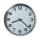How many hours are 15 days?
• Compare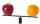Compare with characters >, <, =: 85.57 ? 80.83
• Angles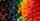Which of those angles are obtuse?
• Valid numberRound the 453874528 on 2 significant numbers.
• Hours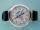How many hours is 9 days?
• To thousands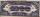Round to thousands following numbers:Added together and write as decimal number: LXVII + MLXIV
• Rounding 2Round the following negative number: -143.021
• Denominator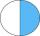Calculate the missing denominator x: ?
• DigitsWrite the smallest and largest 1-digit number.
• Numbers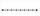Find number on thenumber line that has same distance from this numbers:
• Primes 2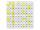Which prime numbers is number 2025 divisible?
• The resultHow many times I decrease the number 1632 to get the result 24?
• Roman numerals 2+Add up the number writtens in Roman numerals. Write the results as a roman numbers.
• Written numberPlace+values x ten thousands =30 thousands
• Roman numerals +Add up the number writtens in Roman numerals. Write the results as a decimal number.
• Plane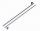On how many parts divide plane 6 parallels?
• Time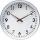16.2 days ..... how many hours is it?
• Roman numeralsWrite numbers written in Roman numerals as decimal.
• MilimetersHow many millimeters is 1/4 meters?

Do you have an interesting mathematical word problem that you can't solve it? Submit a math problem, and we can try to solve it.

We will send a solution to your e-mail address. Solved examples are also published here. Please enter the e-mail correctly and check whether you don't have a full mailbox.

Please do not submit problems from current active competitions such as Mathematical Olympiad, correspondence seminars etc...